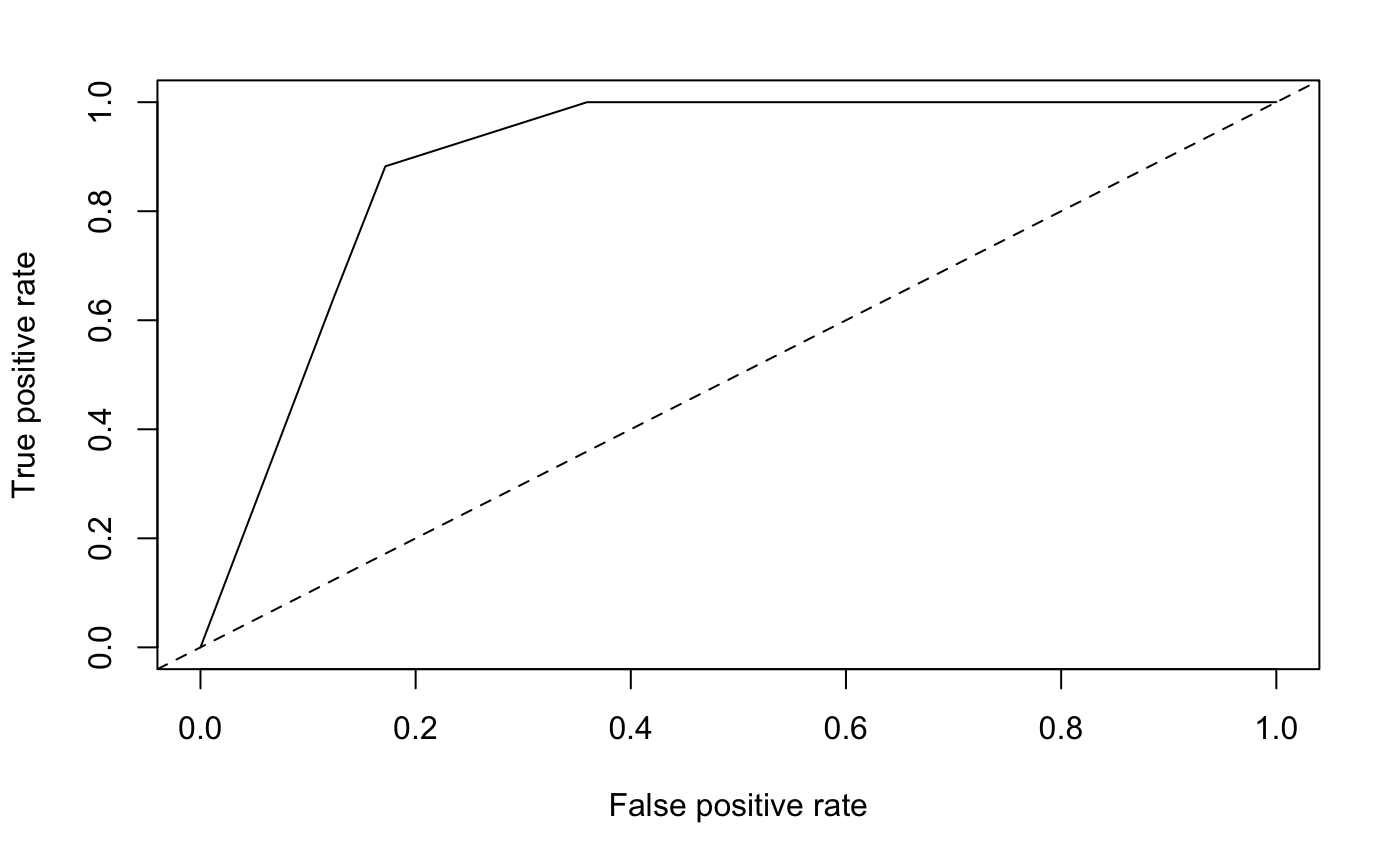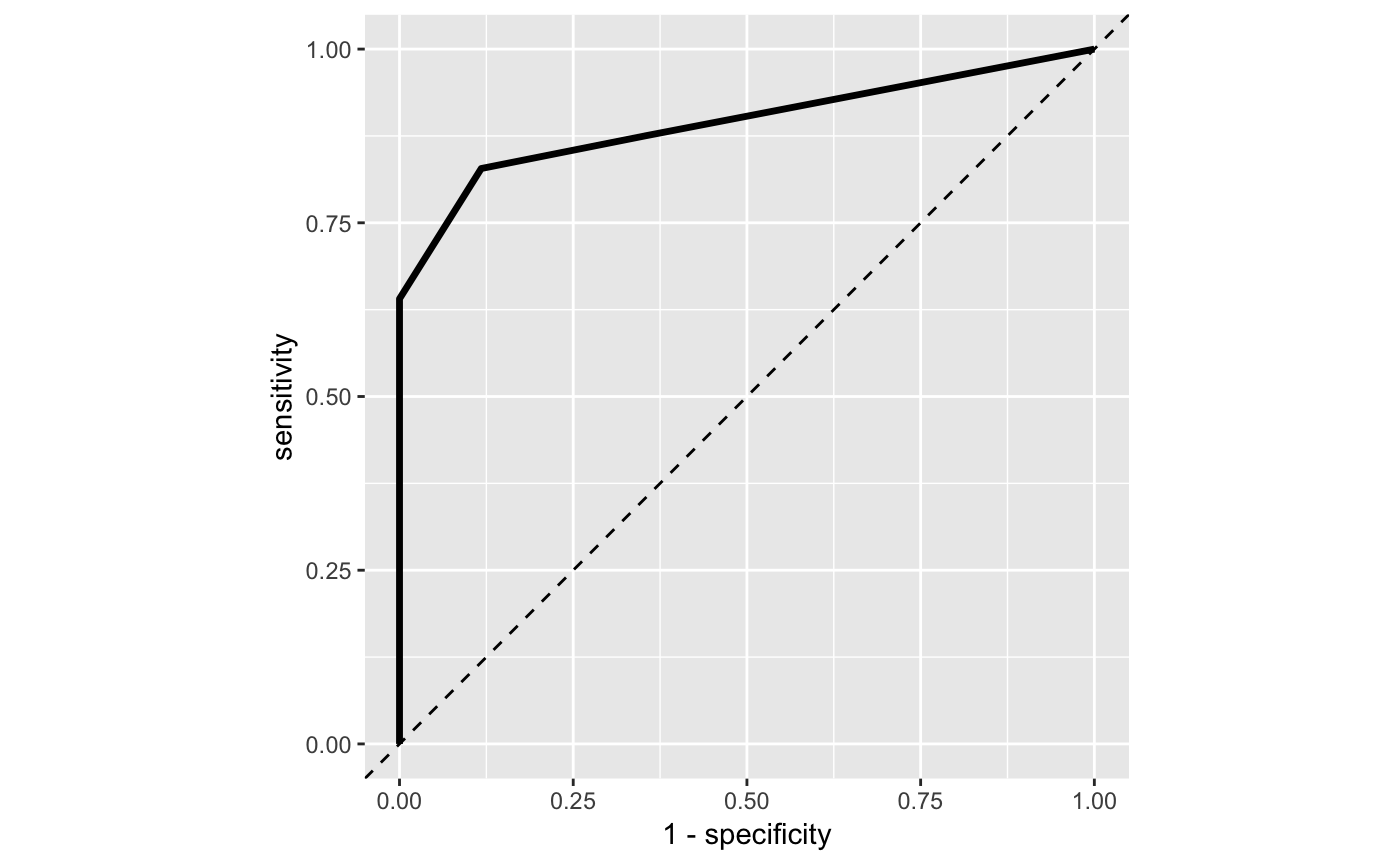# roc_curve() reversed for me #94

Closed
opened this issue Mar 14, 2019 · 2 comments

###igordot commented Mar 14, 2019

 If you are filing a bug, make sure these boxes are checked before submitting your issue— thank you! Start a new R session Install the latest version of of the package: `update.packages(oldPkgs="yardstick", ask=FALSE)` Write a minimal reproducible example run `sessionInfo()` and add the results to the issue. Even better would be to use the `sessioninfo` package's `session_info()`. My `roc_curve()` output plot seems to be flipped, at least compared to the `ROCR` output. ```library("rpart") data("kyphosis", package = "rpart") rp <- rpart(Kyphosis ~ ., data = kyphosis) library("ROCR") pred <- prediction(predict(rp, type = "prob")[, 2], kyphosis\$Kyphosis) plot(performance(pred, "tpr", "fpr")) abline(0, 1, lty = 2)``````library("yardstick") library("ggplot2") pred = data.frame( class1 = predict(rp, type = "prob")[, 1], class2 = predict(rp, type = "prob")[, 2], predicted = predict(rp, type = "class"), truth = kyphosis\$Kyphosis ) roc_tbl = roc_curve(data = pred, truth, class2) ggplot(roc_tbl, aes(x = 1 - specificity, y = sensitivity)) + geom_abline(linetype = 2, slope = 1, intercept = 0) + geom_line(size = 1.2) + theme(aspect.ratio = 1)```Am I doing something wrong? `sessionInfo()`: ``````R version 3.4.4 (2018-03-15) Platform: x86_64-apple-darwin15.6.0 (64-bit) Running under: macOS 10.14.3 Matrix products: default BLAS: /System/Library/Frameworks/Accelerate.framework/Versions/A/Frameworks/vecLib.framework/Versions/A/libBLAS.dylib LAPACK: /Library/Frameworks/R.framework/Versions/3.4/Resources/lib/libRlapack.dylib locale:  en_US.UTF-8/en_US.UTF-8/en_US.UTF-8/C/en_US.UTF-8/en_US.UTF-8 attached base packages:  stats graphics grDevices utils datasets methods base other attached packages:  ggplot2_3.1.0 yardstick_0.0.3 ROCR_1.0-7 gplots_3.0.1 rpart_4.1-13 loaded via a namespace (and not attached):  Rcpp_1.0.0 rstudioapi_0.8 knitr_1.20 magrittr_1.5  munsell_0.4.3 tidyselect_0.2.5 colorspace_1.3-2 R6_2.2.2  rlang_0.3.1 plyr_1.8.4 dplyr_0.8.0.1 caTools_1.17.1  tools_3.4.4 grid_3.4.4 gtable_0.2.0 KernSmooth_2.23-15  withr_2.1.2 gtools_3.5.0 lazyeval_0.2.1 yaml_2.1.18  assertthat_0.2.0 tibble_2.0.1 crayon_1.3.4 purrr_0.2.5  bitops_1.0-6 glue_1.3.1 labeling_0.3 gdata_2.18.0  compiler_3.4.4 pillar_1.3.1 scales_0.5.0 pROC_1.14.0  generics_0.0.2 pkgconfig_2.0.2 ``````

###DavisVaughan commented Mar 14, 2019

 You have to be careful about whether these functions use the first / second level of your factor as the "event". By default, `yardstick` chooses the first level of `truth` as the "event" when computing the roc curve. To alter this, use `options(yardstick.event_first = FALSE)` (this is the pop up you get when you load yardstick). ```library("rpart") library("yardstick") #> For binary classification, the first factor level is assumed to be the event. #> Set the global option `yardstick.event_first` to `FALSE` to change this. library("ggplot2") data("kyphosis", package = "rpart") rp <- rpart(Kyphosis ~ ., data = kyphosis) pred = data.frame( class1 = predict(rp, type = "prob")[, 1], class2 = predict(rp, type = "prob")[, 2], predicted = predict(rp, type = "class"), truth = kyphosis\$Kyphosis ) options(yardstick.event_first = FALSE) roc_tbl = roc_curve(data = pred, truth, class2) ggplot(roc_tbl, aes(x = 1 - specificity, y = sensitivity)) + geom_abline(linetype = 2, slope = 1, intercept = 0) + geom_line(size = 1.2) + theme(aspect.ratio = 1)```Created on 2019-03-14 by the reprex package (v0.2.1.9000)

###igordot commented Mar 14, 2019

 Thank you for pointing out `options(yardstick.event_first = FALSE)`. I didn't notice it because there were other events in the same chunk. Adding that solved the problem. You should consider adding that disclaimer to the function documentation. That setting is already discussed there, but not in the context of binary classification.